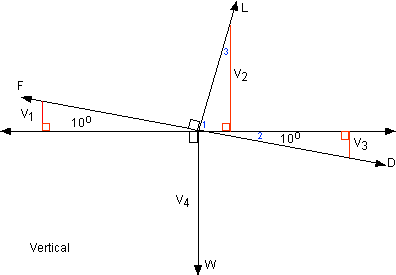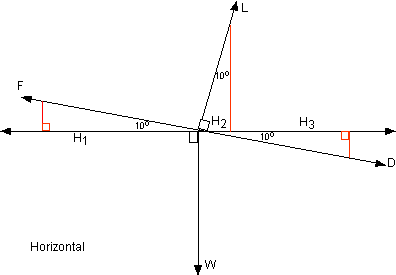+ Text Only Site
+ Non-Flash Version
+ Contact Glenn### Beginner's Guide to Propulsion Forces in a Climb -Vectors Answers

1. Using the first equation: L cos (c) + F sin (c) -D sin (c) - W = ma (vertical)
and the third equation: F -D = Fex , explain and show how to obtain
the fourth equation: L cos (c) + Fex sin (c) - W = ma (vertical).

Factor sin (c) out of last two terms and substitute F
ex for (F - D).

2. Using the second equation and the third equation, explain and show how to obtain the fourth equation.
In the second equation, factor cos (c) out of the first and third terms and substitute as in above.

3.  Why isn't W multiplied by a sine or cosine?
Weight is a downward force, so it would be W sin (90) or just W. (sin 90 is 1).

4. Using the given diagram, theorems/postulates of geometry, and vectors, explain each term in the first two equations.

Vertical Component.1. V1 = F sin 10 positive (upward) vertical

V2 = L cos 10 positive vertical

1. angle 1 + angle 2 = 90 Lift is perpendicular (vertical) to flight path

angle 1 + angle 3 = 90 Acute angles of a right triangle are complementary.

Therefore angle 1 + angle 2 = angle 1 + angle 3

Then angle 2 = angle 3

Since angle 2 = 10 then angle 3 must also.

V3 = D sin 10 negative (downward) vertical

V4 = W

Horizontal Component1. H1 = F cos 10 Positive (forward)

H2 = L sin 10 Negative (backward)

H3 = D cos 10 Negative (backward)

"W" only affects the vertical, not horizontal, or W cos 90 = 0+ Inspector General Hotline + Equal Employment Opportunity Data Posted Pursuant to the No Fear Act + Budgets, Strategic Plans and Accountability Reports + Freedom of Information Act + The President's Management Agenda + NASA Privacy Statement, Disclaimer, and Accessibility CertificationEditor: Tom Benson NASA Official: Tom Benson Last Updated: Thu, May 13 02:38:39 PM EDT 2021 + Contact Glenn